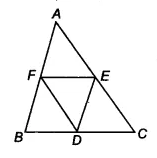Q

# D, E and F are respectively the mid-points of the sides BC, CA and AB of a ∆ ABC. Show that (i) BDEF is a parallelogram.

Q : 5    D, E and F are respectively the mid-points of the sides BC, CA and AB of a  . Show that

(i) BDEF is a parallelogram.

Views

We have a triangle ABC such that D, E and F are the midpoints of the sides BC, CA and AB respectively.Now, in ABC,
F and E are the midpoints of the side AB and AC.
Therefore according to mid-point theorem, the line segment connecting the midpoints of two sides of a triangle is parallel to the third side and half of the third side.
EF || BC or EF || BD

also, EF = 1/2 (BC)
[ D is the mid point of BC]
Similarly, ED || BF and ED = FB
Hence BDEF is a parallelogram.

Exams
Articles
Questions